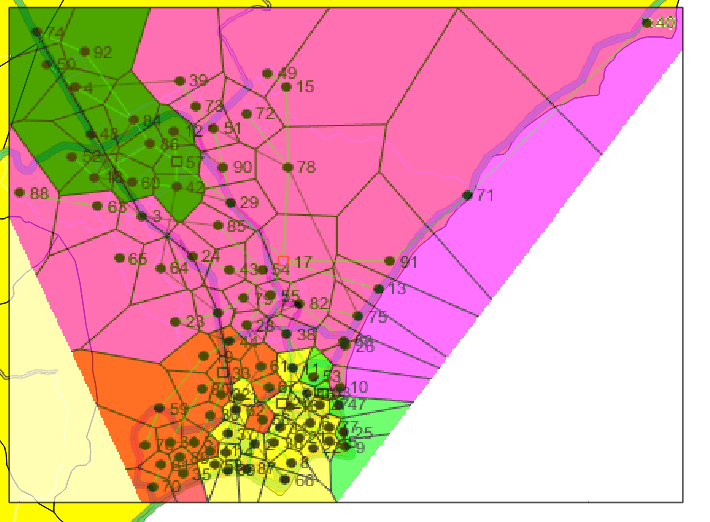# Mobility aware virtual network embedding problem

Post by Inês Ferrão

As a master student, I’m involved in the “MobiWise: from Mobile Sensing to Mobility Advising” project, in collaboration with the Department of Informatics Engineering. In this project, my focus of study is the virtual network embedding problem that consists on mapping virtual network functions to a physical network. More particularly, we are studying the impact of mobility on this mapping.

In a concrete case study, we treat mobile data communications, so the physical network consists of antennas, which have a fixed location at some cell towers, and are connected by a fiber network. To deal with large amounts of data, the current edge computing paradigm, suggests the installation of computational capacity near the end user, thus replacing in some situations the use of a central server, with gains in speed and bandwidth. The question then arises of where to physically place these data centers, and that is where we enter the picture.

In this framework, users’ communications will have to pass between the antenna and a data center, from where they follow to a gateway where they will be directed to some central servers, usually geographically distant. However, as users move, they can change antenna in the middle of an active communication, which can lead to a data center change and the need to pass information between data centers, with the costs associated with it. Thus the optimal position of the data servers is heavily dependent on the movement patterns in the user population.

We mathematically modeled the problem as a variant of the graph homomorphism problem, in which we intend to map a virtual graph to a physical graph, but each edge of the virtual graph can correspond to a path in the physical graph. In Figures 1 and 2, we can see an example of physical network, where nodes are antennas and the edges are fiber connections, and a virtual network, where the edges represent data transmission, and nodes represent data processing by antennas, data centers and gateway.

To solve this problem, we model it as an Integer Linear Program, taking into consideration the capabilities of the edges (bandwidth) and nodes (processing capacity). To illustrate a practical application of the problem in a realistic case, we considered the antennas of the operator Claro S.A., Figure 3, located in the city of Maceió, state of Alagoas, Brazil.Figura 3: Antennas of operator Claro S.A. in the municipality of Maceio, AL, Brazil

Solving the problem for the networks illustrated in Figures 1 and 2, which correspond to this case, and fixing the number of data centers to be installed, we obtain solutions such as the one presented in Figure 4. In this figure, we can visualize, through the different colors, which antennas are served by that data center. We are currently working to improve the various parameters of the model, to better fit it to reality.Figure 4. A possible solution to our problem